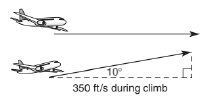Chapter 11.1, Problem 34E### Elementary Geometry for College St...

6th Edition
Daniel C. Alexander + 1 other
ISBN: 9781285195698

#### Solutions

Chapter
Section### Elementary Geometry for College St...

6th Edition
Daniel C. Alexander + 1 other
ISBN: 9781285195698
Textbook Problem
3 views

# In Exercises 27 to 34, use the drawings, where provided, to solve each problem. Angle measures should be given to the nearest degree; distances should be given to the nearest tenth of a unit.An airplane flying at the rate of 350 feet per second begins to climb at an angle of 10◦. What is the increase in altitude over the next 15 seconds?To determine

To find:

The increase in altitude over next 15secs.

Explanation

In a right angle triangle,

1) The side PQ, which is opposite to the right angle PRQ is called the hypotenuse.

(The hypotenuse is the longest side of the right triangle.)

2) The side RQ is called the adjacent side of angle θ.

3) The side PR is called the opposite side of angle θ.

Hence, the sine ratio for an acute angle is oppositehypotenuse

Given:

From, the given diagram,

Angle of elevation is 10

Flying rate = 350 ft/s

Time travel = 15secs

Let a be the increase in altitude over next 15secs which is the opposite side.

Hypotenuse=Flying rate·Time travelling

Hypotenuse=350·15

Hypotenuse = 5250 ft

### Still sussing out bartleby?

Check out a sample textbook solution.

See a sample solution

#### The Solution to Your Study Problems

Bartleby provides explanations to thousands of textbook problems written by our experts, many with advanced degrees!

Get Started

#### In Problems 7-12, find the third derivative.

Mathematical Applications for the Management, Life, and Social Sciences

#### Find all solutions of the equation. 20. x4 = 1

Single Variable Calculus: Early Transcendentals, Volume I

#### Simplify: 728

Elementary Technical Mathematics

#### The length of a = 4i – j – 2k is: 11 21

Study Guide for Stewart's Multivariable Calculus, 8th